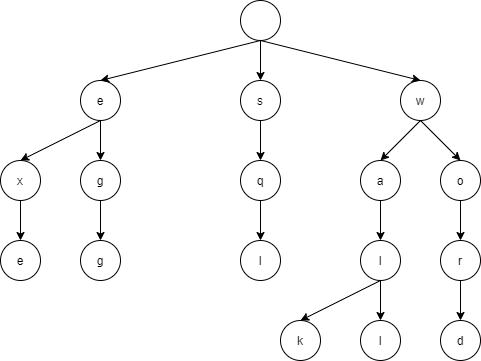# Java implementation of tri-tree

## What is a trie tree?A trie tree is a kind of ordered tree, and it is an algorithm in which characters are stored in each node and a search can be performed by tracing the nodes.

## Write in Java

Create a class that represents a node

``````public class Node {
private int id;
private String str;
private ArrayList<Node> child = new ArrayList<>();
public int getId() {
return this.id;
}
public String getStr() {
return this.str;
}
public ArrayList<Node> getChild(){
return this.child;
}
public void setId(int id) {
this.id = id;
}
public void setStr(String str) {
this.str = str;
}
public void setChild(Node node) {
}
}
``````

The id identifies the node and does not have to be separate str is the character to store in the node child is supposed to store the child of the node

Trees can be built as follows

``````public class Main {
private static ArrayList<Node> nodeList = new ArrayList<>();
private static int id = 1;
public static void insert(Node parent, String word, int charIndex) {
String w = String.valueOf(word.charAt(charIndex));
//System.out.println(w);
if(charIndex == word.length()-1) { //For the last character
for(Node n:parent.getChild()) { //If the last node is an existing node
if(n.getStr() != null&&n.getStr().equals(w)) {
return;
}
}
Node node = new Node();
node.setStr(w);
parent.setChild(node);
node.setChild(node);
node.setId(id);
id += 1;
return;
}
for(Node n:parent.getChild()) { //If the child contains the current ward
if(n.getStr() != null&&n.getStr().equals(w)) {
insert(n,word,charIndex+1);
return;
}
}
Node node = new Node();
node.setStr(w);
parent.setChild(node);
node.setId(id);
id += 1;